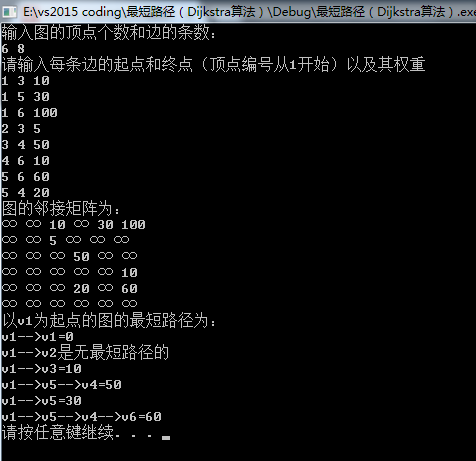Nobody can go back and start a new beginning,but anyone can start today and make a new ending.
Name:Willam
Time:2017/3/8

1、最短路径问题介绍

## 2、Dijkstra算法介绍

• 算法特点：

迪科斯彻算法使用了广度优先搜索解决赋权有向图或者无向图的单源最短路径问题，算法最终得到一个最短路径树。该算法常用于路由算法或者作为其他图算法的一个子模块。

• 算法的思路

Dijkstra算法采用的是一种贪心的策略，声明一个数组dis来保存源点到各个顶点的最短距离和一个保存已经找到了最短路径的顶点的集合：T，初始时，原点 s 的路径权重被赋为 0 （dis[s] = 0）。若对于顶点 s 存在能直接到达的边（s,m），则把dis[m]设为w（s, m）,同时把所有其他（s不能直接到达的）顶点的路径长度设为无穷大。初始时，集合T只有顶点s。
然后，从dis数组选择最小值，则该值就是源点s到该值对应的顶点的最短路径，并且把该点加入到T中，OK，此时完成一个顶点，
然后，我们需要看看新加入的顶点是否可以到达其他顶点并且看看通过该顶点到达其他点的路径长度是否比源点直接到达短，如果是，那么就替换这些顶点在dis中的值。
然后，又从dis中找出最小值，重复上述动作，直到T中包含了图的所有顶点。

## 3、Dijkstra算法示例演示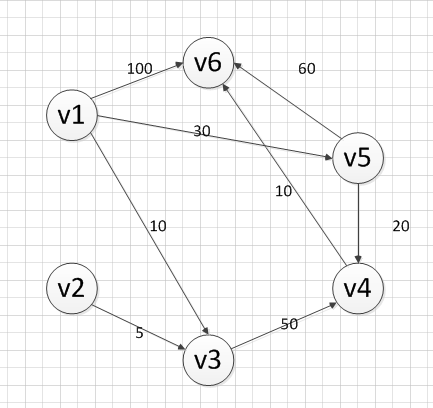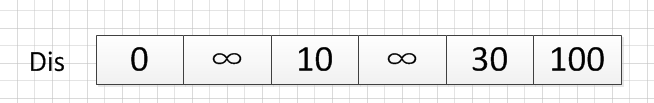OK，既然确定了一个顶点的最短路径，下面我们就要根据这个新入的顶点V3会有出度，发现以v3 为弧尾的有： < v3,v4 >,那么我们看看路径：v1–v3–v4的长度是否比v1–v4短，其实这个已经是很明显的了，因为dis代表的就是v1–v4的长度为无穷大，而v1–v3–v4的长度为：10+50=60，所以更新dis的值,得到如下结果：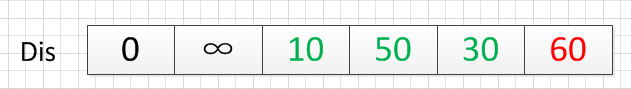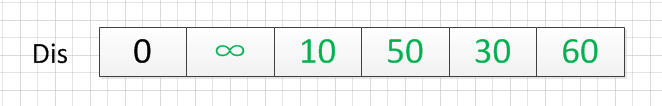``````起点  终点    最短路径    长度
v1    v2     无          ∞
v3     {v1,v3}    10
v4     {v1,v5,v4}  50
v5     {v1,v5}    30
v6     {v1，v5,v4,v6} 60
``````

## 4、Dijkstra算法的代码实现（c++）

• Dijkstra.h文件的代码
``````/************************************************************/
/*                程序作者：Willam                          */
/*                程序完成时间：2017/3/8                    */
/*                有任何问题请联系：2930526477@qq.com       */
/************************************************************/
//@尽量写出完美的程序

#pragma once
//#pragma once是一个比较常用的C/C++杂注，
//只要在头文件的最开始加入这条杂注，
//就能够保证头文件只被编译一次。

#include<iostream>
#include<string>
using namespace std;

/*

*/
//记录起点到每个顶点的最短路径的信息
struct Dis {
string path;
int value;
bool visit;
Dis() {
visit = false;
value = 0;
path = "";
}
};

class Graph_DG {
private:
int vexnum;   //图的顶点个数
int edge;     //图的边数
int **arc;   //邻接矩阵
Dis * dis;   //记录各个顶点最短路径的信息
public:
//构造函数
Graph_DG(int vexnum, int edge);
//析构函数
~Graph_DG();
// 判断我们每次输入的的边的信息是否合法
//顶点从1开始编号
bool check_edge_value(int start, int end, int weight);
//创建图
void createGraph();
//打印邻接矩阵
void print();
//求最短路径
void Dijkstra(int begin);
//打印最短路径
void print_path(int);
};
``````
• Dijkstra.cpp文件的代码
``````#include"Dijkstra.h"

//构造函数
Graph_DG::Graph_DG(int vexnum, int edge) {
//初始化顶点数和边数
this->vexnum = vexnum;
this->edge = edge;
//为邻接矩阵开辟空间和赋初值
arc = new int*[this->vexnum];
dis = new Dis[this->vexnum];
for (int i = 0; i < this->vexnum; i++) {
arc[i] = new int[this->vexnum];
for (int k = 0; k < this->vexnum; k++) {
//邻接矩阵初始化为无穷大
arc[i][k] = INT_MAX;
}
}
}
//析构函数
Graph_DG::~Graph_DG() {
delete[] dis;
for (int i = 0; i < this->vexnum; i++) {
delete this->arc[i];
}
delete arc;
}

// 判断我们每次输入的的边的信息是否合法
//顶点从1开始编号
bool Graph_DG::check_edge_value(int start, int end, int weight) {
if (start<1 || end<1 || start>vexnum || end>vexnum || weight < 0) {
return false;
}
return true;
}

void Graph_DG::createGraph() {
cout << "请输入每条边的起点和终点（顶点编号从1开始）以及其权重" << endl;
int start;
int end;
int weight;
int count = 0;
while (count != this->edge) {
cin >> start >> end >> weight;
//首先判断边的信息是否合法
while (!this->check_edge_value(start, end, weight)) {
cout << "输入的边的信息不合法，请重新输入" << endl;
cin >> start >> end >> weight;
}
//对邻接矩阵对应上的点赋值
arc[start - 1][end - 1] = weight;
//无向图添加上这行代码
//arc[end - 1][start - 1] = weight;
++count;
}
}

void Graph_DG::print() {
cout << "图的邻接矩阵为：" << endl;
int count_row = 0; //打印行的标签
int count_col = 0; //打印列的标签
//开始打印
while (count_row != this->vexnum) {
count_col = 0;
while (count_col != this->vexnum) {
if (arc[count_row][count_col] == INT_MAX)
cout << "∞" << " ";
else
cout << arc[count_row][count_col] << " ";
++count_col;
}
cout << endl;
++count_row;
}
}
void Graph_DG::Dijkstra(int begin){
//首先初始化我们的dis数组
int i;
for (i = 0; i < this->vexnum; i++) {
//设置当前的路径
dis[i].path = "v" + to_string(begin) + "-->v" + to_string(i + 1);
dis[i].value = arc[begin - 1][i];
}
//设置起点的到起点的路径为0
dis[begin - 1].value = 0;
dis[begin - 1].visit = true;

int count = 1;
//计算剩余的顶点的最短路径（剩余this->vexnum-1个顶点）
while (count != this->vexnum) {
//temp用于保存当前dis数组中最小的那个下标
//min记录的当前的最小值
int temp=0;
int min = INT_MAX;
for (i = 0; i < this->vexnum; i++) {
if (!dis[i].visit && dis[i].value<min) {
min = dis[i].value;
temp = i;
}
}
//cout << temp + 1 << "  "<<min << endl;
//把temp对应的顶点加入到已经找到的最短路径的集合中
dis[temp].visit = true;
++count;
for (i = 0; i < this->vexnum; i++) {
//注意这里的条件arc[temp][i]!=INT_MAX必须加，不然会出现溢出，从而造成程序异常
if (!dis[i].visit && arc[temp][i]!=INT_MAX && (dis[temp].value + arc[temp][i]) < dis[i].value) {
//如果新得到的边可以影响其他为访问的顶点，那就就更新它的最短路径和长度
dis[i].value = dis[temp].value + arc[temp][i];
dis[i].path = dis[temp].path + "-->v" + to_string(i + 1);
}
}
}

}
void Graph_DG::print_path(int begin) {
string str;
str = "v" + to_string(begin);
cout << "以"<<str<<"为起点的图的最短路径为：" << endl;
for (int i = 0; i != this->vexnum; i++) {
if(dis[i].value!=INT_MAX)
cout << dis[i].path << "=" << dis[i].value << endl;
else {
cout << dis[i].path << "是无最短路径的" << endl;
}
}
}``````
• main.cpp文件的代码
``````#include"Dijkstra.h"

//检验输入边数和顶点数的值是否有效，可以自己推算为啥：
//顶点数和边数的关系是：((Vexnum*(Vexnum - 1)) / 2) < edge
bool check(int Vexnum, int edge) {
if (Vexnum <= 0 || edge <= 0 || ((Vexnum*(Vexnum - 1)) / 2) < edge)
return false;
return true;
}
int main() {
int vexnum; int edge;

cout << "输入图的顶点个数和边的条数：" << endl;
cin >> vexnum >> edge;
while (!check(vexnum, edge)) {
cout << "输入的数值不合法，请重新输入" << endl;
cin >> vexnum >> edge;
}
Graph_DG graph(vexnum, edge);
graph.createGraph();
graph.print();
graph.Dijkstra(1);
graph.print_path(1);
system("pause");
return 0;
}``````

``````6 8
1 3 10
1 5 30
1 6 100
2 3 5
3 4 50
4 6 10
5 6 60
5 4 20``````